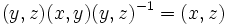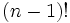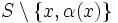# Isotropy of a point has double coset index two in finitary symmetric group

This article gives the statement, and possibly proof, of a particular subgroup or type of subgroup satisfying a particular subgroup property (namely, Subgroup of double coset index two (?)) in a particular group or type of group (namely, Finitary symmetric group (?)).

## Statement

Suppose$S$ is a set of size at least two. Let$G = \operatorname{FSym}(S)$ be the finitary symmetric group on$S$. Let$x \in S$ and$H$ denote the subgroup of$G$ comprising those permutations that fix$x$; in other words,$H$ is the isotropy subgroup of$x$ and equivalently$H$ is the finitary symmetric group on$S \setminus \{ x \}$.

Then,$H$ has double coset index two in$G$.

## Related facts

• Isotropy of a point has double coset index two in symmetric group: The analogous result holds even when we are considering arbitrary permutations, i.e., permutations that are not necessarily finitary.
• Isotropy of a point has double coset index two in finitary alternating group
• Isotropy of finite subset has finite double coset index in symmetric group: If a subset has size$n$, then the double coset index of its isotropy subgroup is bounded by a function of$n$. In fact, if the whole set has size at least$2n$, the double coset index is precisely equal to that function of$n$.
• Isotropy of finite subset has finite double coset index in finitary symmetric group: If a subset has size$n$, then the double coset index of its isotropy subgroup is bounded by a function of$n$. In fact, if the whole set has size at least$2n$, the double coset index is precisely equal to that function of$n$.
• Isotropy of finite subset has finite double coset index in finitary alternating group: If a subset has size$n$, then the double coset index of its isotropy subgroup is bounded by a function of$n$. In fact, if the whole set has size at least$2n$, the double coset index is precisely equal to that function of$n$.

## Facts used

1. Product formula

## Proof

### Direct proof

Given:$S$ is a set of size at least two,$G = \operatorname{FSym}(S)$,$H$ is the isotropy subgroup of$x$.

To prove:$H$ has double coset index two in$G$.

Proof:

1. The transpositions$(x,y)$ (along with the identity element) form a system of left coset representatives for$H$ in$G$: Indeed, given any finitary permutation$\sigma \notin H$, we have$\sigma(x) \ne x$. Define$\tau = (x,\sigma(x))$. The permutation$\tau^{-1}\sigma$ fixes$x$, so$\sigma \in \tau H$. Thus, every element of$G$ outside$H$ can be expressed in the form$(x,y)H$ for some$y$, so every left coset contains a transposition of the form$(x,y)$. Further, for$y \ne z$, we have that$(x,y)^{-1}(x,z)$ is not in$H$, so distinct transpositions correspond to different left cosets of$H$ in$G$. (Note that the left coset representative for$H$ itself is the identity element).
2. The transpositions$(x,y)$ and$(x,z)$ are conjugate via$(y,z)$. In particular, they are conjugate via$H$: In other words,$(y,z)(x,y)(y,z)^{-1} = (x,z)$.
3. Pick any$y \ne x$. We claim that if$\alpha = (x,y)$, then$G = H \cup H\alpha H$: Pick any$\sigma \notin H$. By step (1),$\sigma \in \tau H$ for some$\tau = (x,z)$. If$y = x$,$\sigma \in \alpha H$ and we are done. Else, by step (2), we have$\tau = (y,z)(x,y)(y,z)^{-1} \in H(x,y)H$. Thus,$\sigma \in \tau H \subseteq H \alpha H$, completing the proof.

### Proof by size computations

(This proof works only for finite sets).

Given: A finite set$S$ of size$n \ge 2$.$G$ is the symmetric group on$S$ and$H$ is the subgroup comprising permutations that fix a particular point$x \in S$.

To prove:$H$ has double coset index two.

Proof: We need to prove that for$\alpha \in G \setminus H$, we have$|H \alpha H| = |G \setminus H|$. We know that:$|H \alpha H| = |H \alpha H \alpha^{-1}| = |H (\alpha H \alpha^{-1})| = \frac{|H||\alpha H \alpha^{-1}|}{|H \cap \alpha H \alpha^{-1}|}$.

(The last step is from the product formula, fact (1).

Now, note that for any$\alpha \notin H$,$\alpha H \alpha^{-1}$ is the isotropy subgroup of$\alpha(x)$. Hence:

•$G$ has size$n!$.
• Both$H$ and$\alpha H \alpha^{-1}$ are subgroups of size$(n-1)!$: They are the symmetric groups on the sets$S \setminus \{ x \}$ and$S \setminus \{ \alpha(x) \}$ respectively.
• The intersection is a subgroup of size$(n-2)!$: It is the symmetric group on the set$S \setminus \{ x, \alpha(x)\}$.

Plugging in the formula yields:$|H\alpha H| = \frac{(n-1)!^2}{(n-2)!}$.

This simplifies to:$|H\alpha H| = (n-1)(n-1)! = n! - (n-1)! = |G \setminus H|$.

Cardinality considerations thus yield:$H \alpha H = G \setminus H$.

This completes the proof.